# Combining bootstrapping with multiple imputation

Multiple imputation (MI) is a popular approach to handling missing data. In the final part of MI, inferences for parameter estimates are made based on simple rules developed by Rubin. These rules rely on the analyst having a calculable standard error for their parameter estimate for each imputed dataset. This is fine for standard analyses, e.g. regression models fitted by maximum likelihood, where standard errors based on asymptotic theory are easily calculated. However, for many analyses analytic standard errors are not available, or are prohibitive to find by analytical methods. For such methods, if there were no missing data, an attractive approach for finding standard errors and confidence intervals is the method of bootstrapping. However, if one is using MI to handle missing data, and would ordinarily use bootstrapping to find standard errors / confidence intervals, how should these be combined?

A very nice paper recently submitted to arXiv, by Schomaker & Heumann investigates this question. They consider a number of possible ways of combining bootstrapping and MI. The two main approaches are either to first impute missing data, and then use bootstrapping to obtain an estimate of the within-imputation SE for each imputed dataset, or, to bootstrap the original data, and apply MI separately to each bootstrapped dataset. The MI point estimate found from each bootstrap is then used to construct bootstrap SEs and confidence intervals in the usual way.

Through both simulation and theoretical considerations, Schomaker & Heumann demonstrate that one should use the latter approach: i.e. to bootstrap the original data, and apply MI to each of the bootstrapped datasets. In contrast, the approach which uses bootstrapping to find a SE for each imputed dataset results in SEs which are much too large and confidence intervals which over cover. I think this is a very valuable investigation and paper, and recommend those who need to combine bootstrapping with MI to read it.

One aspect I cannot quite understand is their explanation for the reason that MI followed by bootstrapping (MI boot) doesn’t work. On page 11 they state (correctly I think) that applying the bootstrap to the m’th imputed dataset estimates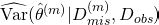, the variance of the m’th imputed point estimate of theta, conditional on the observed data and the imputed values in the m’th imputation – i.e. the within-imputation variance of theta. They then write

These estimates are not identical to the variance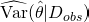which we need to apply (3.2). Combining the M estimates is not meaningful because the quantity we use in our calculation is not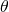but rather M different quantities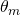which are all not unconditional on the missing and imputed data.

I don’t really understand this –is the total posterior variance of, which is what we want to estimate with Rubin’s rules. But the within-imputation variance is supposed to be the average (across the predictive distribution of the missing data) posterior variance ofconditional on the imputed and observed data. Thinking of it very directly, for a given set of imputed+observed data, the ‘MI boot’ approach ought to work if the bootstrap SE for that imputed dataset is close to what the analytical SE is (were we able to calculate it). And I can’t see why this wouldn’t be the case. If anyone can explain this to me, please add a comment!

27th July 2016 – postscript – please see the comments below, in particular from the authors and R code to perform the simulation study.

22nd February 2017 – Schomaker & Heumann have put a revised version of their paper online, available here. I haven’t read it yet, but I believe they may resolved my query above.

2019 – the paper is now published in Statistics in Medicine

### 15 thoughts on “Combining bootstrapping with multiple imputation”

1.Any suggestions about how this approach (i.e., bootstrapping followed by multiple imputation) might be accomplished using SPSS?

•Sorry but I’m not very familiar with SPSS these days. But in principle it ought not to be tricky: 1) manually generate m bootstrap resampled datasets. 2) apply MI to each of these bootstrap datasets, which results in point estimates from Rubin’s rules 3) obtain bootstrap inferences from the m estimates using bootstrap methods as usual. e.g. the bootstrap SE would be the standard deviation of the m estimates across the m bootstrap samples. Hope that helps.

2.Hi Jonathan – I was of your colleagues at the London School. I’d like to try this out, though in my situation I reckon the use of bootstrapping and MI are overkill (<5% missing data on a potential confounder). But it's a low-stakes chance for me to try something new.

By chance can you point me to any Stata code for this procedure? I am able to run my linear regression model with MI for the potential confounder. And separately I am able to write a program to bootstrap the linear regression model with complete-case data. But I get an error when I try to nest MI into my bootstrap.

•Hi Sujit. No I haven’t got any code to give/point you to I’m afraid. But what you are trying to do ought to work. I guess your program that performs the MI is no returning the results or not leaving the dataset in the form that the bootstrap expects it to. If you can’t get it work I would suggest posting to the Stata List forum.

•Jonathan is correct, the Bootstrap expects the mi data to be set to wide format:

mi set wide

It should work fine once you do this (provided your Bootstrap program that performs the MI is working).

3.Hi Jonathan. Thanks for your interest in our paper and the interesting blog. We had observed the problem of constructing bootstrap confidence intervals under MI many times, and it is encouraging to see that our research provides some guidance to you and others.

In fact, it was interesting to us as well that in almost all of our simulations and data examples confidence intervals were far too large when first multiply imputing and then bootstrapping. However, as you see in the last data example there exist some situations were there is not much difference between impute-bootstrap and bootstrap-impute, it depends on the within imputation, between imputation, within bootstrap, and between bootstrap variance of the data set of interest,

So, why is it nevertheless most often the case that bootstrapping imputed data gives us these problems? Intuitively the answer is that you bootstrap imputed data which contains both sampling and imputation uncertainty, and you do this M times for each bootstrap sample. Each bootstraped data set contains additional imputation uncertainty and varies with respect to the m-th imputation. In our theoretical considerations we try to motivate this by looking on what MI does and which parameters are estimated.It is not trivial, and really a bit tricky, but essentially with MI followed by bootstrap you don’t estimate theta (the parameter of interest of the analysis model) anymore, but something different. Have again a look at formula (7) and the text below. Again, intuitively, think of the 1st imputed data set. This data set contains both sampling and imputation uncertainty. Now you bootstrap it. You use the bootstrap samples to estimate the standard error of theta. What you do in fact is to estimate the standard error of theta^m, i.e the the m-th imputation estimate of theta (i.e. theta^m not theta) that contains the imputation uncertainty of this specific imputed dataset. If you find this issue still confusing (which it is), and you are still interested in the details, we can send you the “simple” simulation setting where you can easily see what exactly happens and you can play with it.

For the others: we have done everything in R where you can boostrap the data, for example using the “sample” command, then impute with amelia (or mice or other options), then combine results using library norm (or Zelig or by hand) and then store the results in a matrix.

For those who don’t use R, it is easier to implement bootstrapping and MI in Stata than SPSS. Stata has a good chained equation imputation command (“ice”) and allows for bootstrapping (“bootstrap”). I would say, if you have some basic ideas about programming in R or Stata it will only be a few lines to implement the approach but there is no ready-made package/macro that does it automatically (except my R-package in the particular context of model selection/averaging: https://r-forge.r-project.org/R/?group_id=2152).

Cheers
Michael

•Thanks for the reply Michael. I’m afraid I still don’t follow the logic. On p11, in relation to MI boot, you say that:

The variance estimates obtained from the imputed datasets using bootstrapping are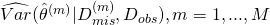and are therefore conditional on the mth imputation draw. These estimates are not identical to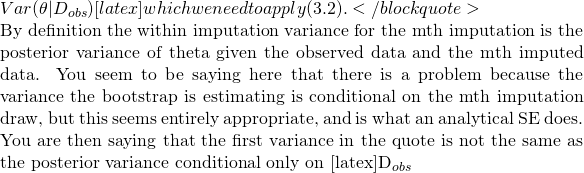, which I agree with, but we are only using the bootstrap to estimate the within-imputation (effectively a full data) posterior variance, not the observed data posterior variance.

I couldn’t resist investigating myself, and so I attempted to reproduce your first simulation study. The only difference I think is that I used 100 bootstrap samples rather than 200. I include my code below. For beta1, the average across 1,000 simulations of the Rubin’s rules variance estimator based on the analytical within-imputation variance is almost identical to the Rubin’s rule variance estimator which uses bootstrapping to estimate the within-imputation variance. This seems to contrast starkly with your results, where the MI Boot SE four times the size of the one based on the analytical within-imputation variance. Perhaps we have done something differently?

library(mice)
library(boot)

fitoutmodel <- function(data,indices) {
localdata <- data[indices,]
mod <- lm(y~x1, data=localdata)
coef(mod)
}

m <- 10
nSim <- 1000

miEsts <- array(0, dim=nSim)
analyticVar <- array(0, dim=nSim)
bootVar <- array(0, dim=nSim)

for (sim in 1:nSim) {
print(sim)

n <- 1000
x1 <- rnorm(n)
y <- 0.4*x1 + rnorm(n, sd=2^0.5)

pi <- 1-1/((0.25*y)^2 +1)
r <- 1*(runif(n)>pi)
x1[r==0] <- NA

obsdata <- data.frame(x1,y)

imps <- mice(data=obsdata,method="norm",m=m,printFlag = F)

impdatasets <- list(m)

impEsts <- array(0, dim=m)
analyticSE <- array(0, dim=m)
bootSE <- array(0, dim=m)

for (i in 1:m) {
impdatasets[[i]] <- complete(imps,i)

impmod <- lm(y~x1, data=impdatasets[[i]])
impEsts[i] <- coef(impmod)
analyticSE[i] <- vcov(impmod)[2,2]^0.5

boot <- boot(impdatasets[[i]],fitoutmodel,R=100)
bootSE[i] <- sd(boot\$t)
}

miEsts[sim] <- mean(impEsts)
analyticVar[sim] <- mean(analyticSE^2)+var(impEsts)
bootVar[sim] <- mean(bootSE^2)+var(impEsts)

}

sd(miEsts)
mean(analyticVar^0.5)
mean(bootVar^0.5)

> sd(miEsts)
 0.05234075
> mean(analyticVar^0.5)
 0.05025574
> mean(bootVar^0.5)
 0.05022578
•Hi Jonathan,

thanks for working through the simulations and engaging with the subject matter; it is fantastic that we receive detailed feedback on the topic and there is so much interest in it. So, it was definitely worth posting the article on arxiv before publication.

The brief answer is: I browsed through your code and couldn’t find an obvious error. I then checked the data example from us, i.e. our initial motivation where I asked myself what to do, and I couldn’t find an error either. I then went step by step through my simulation code again, and eventually I discovered a tiny but relevant error. I am currently on long leave, and away for most of August/September – but after that I’ll rerun the simulations and check the conclusions again.They may change, as you say. I’ll keep you updated towards the end of the year when we have an updated summary….

Have a great summer

Michael

•Hi Michael and Jonathan. It looks like I came to the discussion 6 years to late. I have been applying Michael’s paper to a consulting problem using MI boot (pooled). Following Shah’s well known paper, I implemented the BCa method for bootstrap confidence intervals for the case with no missing values. I would prefer to extend the BCa method to the MI boot (pooled) case, but I cannot find any papers in the literature that have researched it, which gives me pause. I do see a possible problem with trying to average acceleration and bias constants across imputation sets, because their expected values are sample size dependent. I wondering whether I could apply the BCa method to the pooled bootstraps. I would love to hear from the two of you any advice on how to implement the BCa method to the missing value case or reasons why that would not be a good idea. Thank you advance for your consideration.
Best,
Brian

4.Are there any updates error in the codes? I’m currently doing a research on this and it will greatly help us.

Thank you so much!

5.Hi, you can find the most recent version of the article, included updated results, here:
https://arxiv.org/abs/1602.07933
[currently version v4]
I hope it helps,
Best wishes
Michael

6.Schomaker and Heumann’s paper has now been published in Statistics in Medicine: https://doi.org/10.1002/sim.7654

7.Hi,
Do you have a SAS code for BOOT MI or MI BOOT as opposed by Schomaker & Heumann?

Thank you very much,
Laura

•